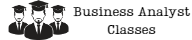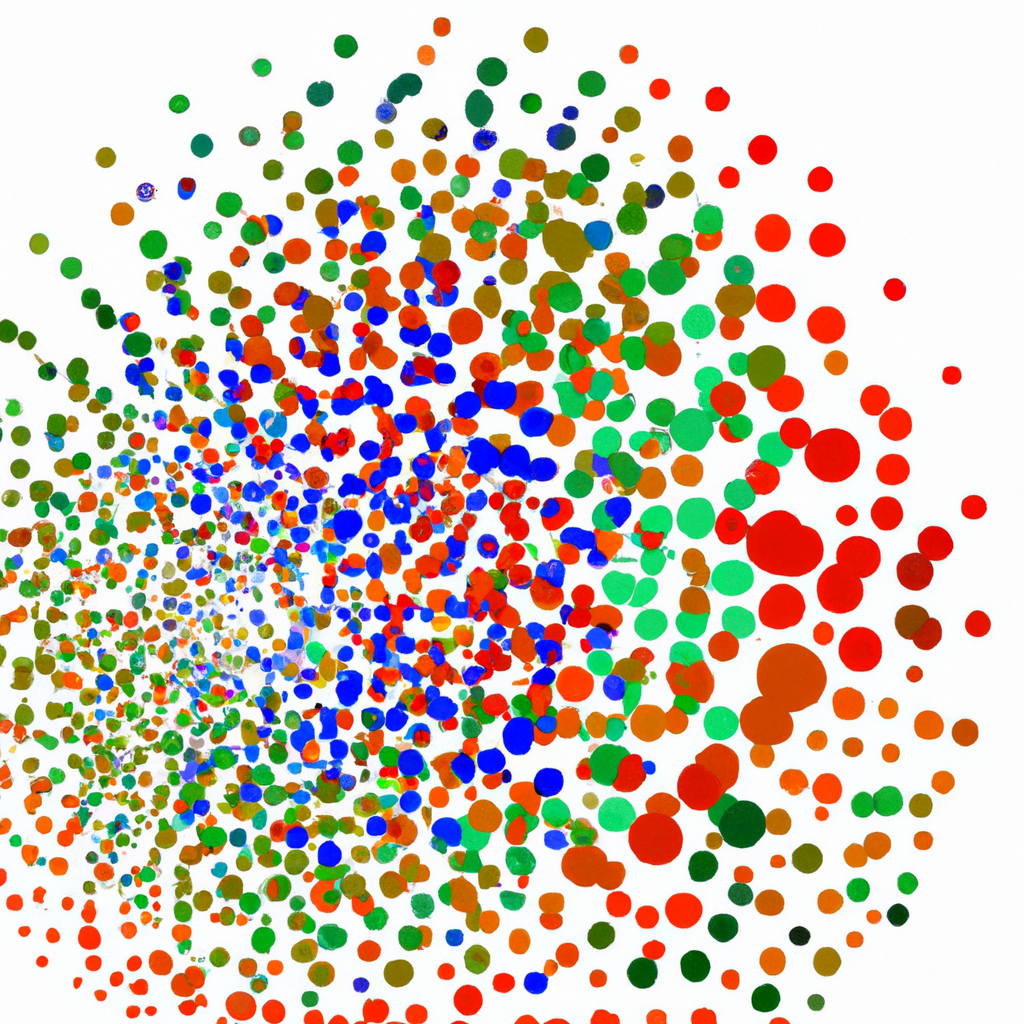# How to Use Principal Component Analysis to Simplify Complex Data

As organizations gather and store more data, it becomes increasingly difficult to analyze and make sense of it all. In many cases, the data is complex, with numerous variables that need to be considered. This is where principal component analysis (PCA) comes in.PCA is a statistical technique that simplifies complex data by reducing the number of variables while preserving as much of the original data’s variation as possible. In this article, we will explain what principal component analysis is, how it works, and how it can be used to simplify complex data.

## What is Principal Component Analysis?

Principal component analysis is a mathematical technique that transforms a set of correlated variables into a smaller set of uncorrelated variables, called principal components. The principal components are linear combinations of the original variables, and they capture as much of the original data’s variation as possible.

PCA is particularly useful when dealing with high-dimensional data, where the number of variables is much larger than the number of observations. In such cases, PCA can help to reduce the dimensionality of the data, making it easier to visualize and analyze.

## How Does Principal Component Analysis Work?

PCA works by identifying the directions in which the data varies the most. These directions are called principal components, and they are orthogonal to each other, meaning that they are uncorrelated.

The first principal component is the direction of maximum variation in the data. The second principal component is the direction of maximum variation that is orthogonal to the first principal component, and so on. Each principal component captures a decreasing amount of the remaining variation in the data.

To compute the principal components, PCA uses a technique called singular value decomposition (SVD). SVD decomposes the data matrix into three matrices: U, Σ, and V. The columns of U are the eigenvectors of the covariance matrix of the data, and the columns of V are the eigenvectors of the covariance matrix of the transposed data. The diagonal elements of Σ are the singular values of the data matrix.

## How to Use Principal Component Analysis

PCA can be used in a variety of applications, including exploratory data analysis, data visualization, and machine learning. Here are some examples of how PCA can be used:

### Exploratory Data Analysis

PCA can be used to explore the relationships between variables in a dataset. By visualizing the principal components, we can identify patterns and clusters in the data that are not immediately apparent from the raw data.

### Data Visualization

PCA can be used to project high-dimensional data onto a lower-dimensional space, making it easier to visualize and interpret. For example, if we have a dataset with 100 variables, we can use PCA to project the data onto a 2D or 3D space, which can be easily plotted.

### Machine Learning

PCA can be used as a preprocessing step in machine learning algorithms to reduce the dimensionality of the data. By reducing the number of variables, we can improve the performance of the machine learning algorithm and reduce the risk of overfitting.

## Conclusion

Principal component analysis is a powerful tool for simplifying complex data. By reducing the number of variables while preserving as much of the original data’s variation as possible, PCA can help to identify patterns and clusters in the data that are not immediately apparent from the raw data. PCA can be used in a variety of applications, including exploratory data analysis, data visualization, and machine learning. By understanding how PCA works and how to use it, you can gain deeper insights into your data and make more informed decisions.

### Try some of our classes

Enter your email and we’ll send you some samples of our favorite classes.

#### Course Categories

Quisque velit nisi, pretium ut lacinia in, elementum id enim.